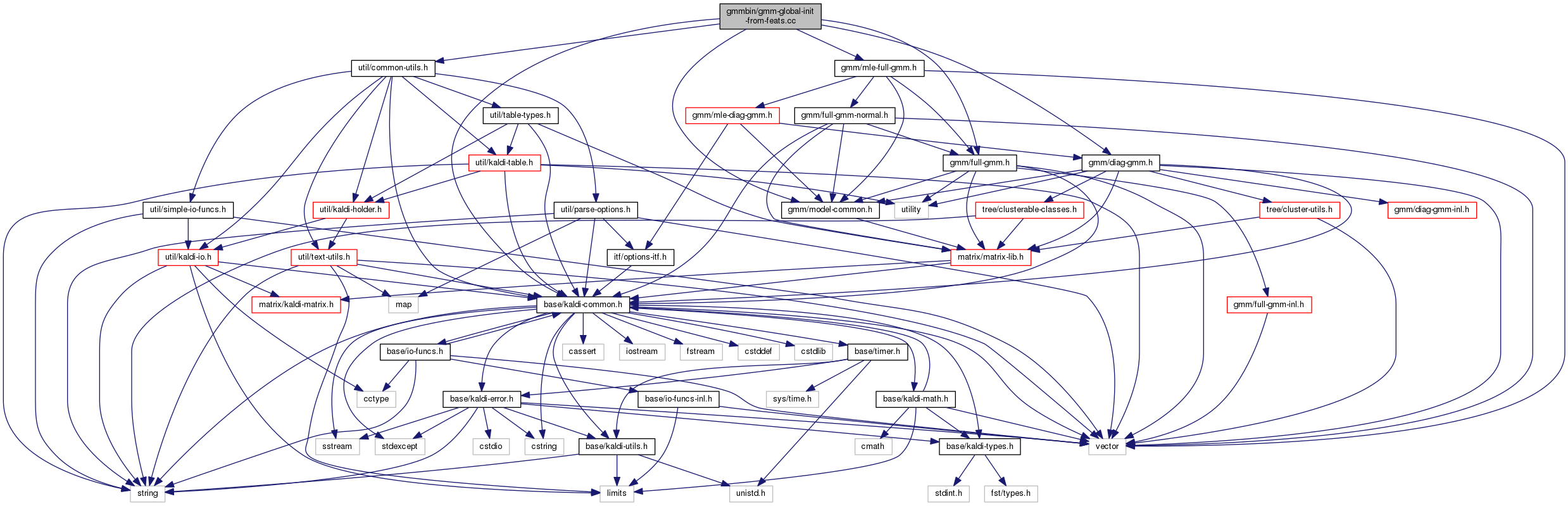gmm-global-init-from-feats.cc File Reference
`#include "base/kaldi-common.h"`
`#include "util/common-utils.h"`
`#include "gmm/model-common.h"`
`#include "gmm/full-gmm.h"`
`#include "gmm/diag-gmm.h"`
`#include "gmm/mle-full-gmm.h"`
Include dependency graph for gmm-global-init-from-feats.cc:Go to the source code of this file.

## Namespaces

kaldi
This code computes Goodness of Pronunciation (GOP) and extracts phone-level pronunciation feature for mispronunciations detection tasks, the reference:

## Functions

void InitGmmFromRandomFrames (const Matrix< BaseFloat > &feats, DiagGmm *gmm)

void TrainOneIter (const Matrix< BaseFloat > &feats, const MleDiagGmmOptions &gmm_opts, int32 iter, int32 num_threads, DiagGmm *gmm)

int main (int argc, char *argv[])

## ◆ main()

 int main ( int argc, char * argv[] )

Definition at line 88 of file gmm-global-init-from-feats.cc.

88  {
89  try {
90  using namespace kaldi;
91
92  const char *usage =
93  "This program initializes a single diagonal GMM and does multiple iterations of\n"
94  "training from features stored in memory.\n"
95  "Usage: gmm-global-init-from-feats [options] <feature-rspecifier> <model-out>\n"
96  "e.g.: gmm-global-init-from-feats scp:train.scp 1.mdl\n";
97
98  ParseOptions po(usage);
99  MleDiagGmmOptions gmm_opts;
100
101  bool binary = true;
102  int32 num_gauss = 100;
103  int32 num_gauss_init = 0;
104  int32 num_iters = 50;
105  int32 num_frames = 200000;
106  int32 srand_seed = 0;
108
109  po.Register("binary", &binary, "Write output in binary mode");
110  po.Register("num-gauss", &num_gauss, "Number of Gaussians in the model");
111  po.Register("num-gauss-init", &num_gauss_init, "Number of Gaussians in "
112  "the model initially (if nonzero and less than num_gauss, "
113  "we'll do mixture splitting)");
114  po.Register("num-iters", &num_iters, "Number of iterations of training");
115  po.Register("num-frames", &num_frames, "Number of feature vectors to store in "
116  "memory and train on (randomly chosen from the input features)");
117  po.Register("srand", &srand_seed, "Seed for random number generator ");
119  "statistics accumulation");
120
121  gmm_opts.Register(&po);
122
124
125  srand(srand_seed);
126
127  if (po.NumArgs() != 2) {
128  po.PrintUsage();
129  exit(1);
130  }
131
132  std::string feature_rspecifier = po.GetArg(1),
133  model_wxfilename = po.GetArg(2);
134
135  Matrix<BaseFloat> feats;
136
138
139
140  KALDI_ASSERT(num_frames > 0);
141
142  int64 num_read = 0, dim = 0;
143
144  KALDI_LOG << "Reading features (will keep " << num_frames << " frames.)";
145
147  const Matrix<BaseFloat> &this_feats = feature_reader.Value();
148  for (int32 t = 0; t < this_feats.NumRows(); t++) {
150  if (dim == 0) {
151  dim = this_feats.NumCols();
152  feats.Resize(num_frames, dim);
153  } else if (this_feats.NumCols() != dim) {
154  KALDI_ERR << "Features have inconsistent dims "
155  << this_feats.NumCols() << " vs. " << dim
156  << " (current utt is) " << feature_reader.Key();
157  }
158  if (num_read <= num_frames) {
160  } else {
161  BaseFloat keep_prob = num_frames / static_cast<BaseFloat>(num_read);
162  if (WithProb(keep_prob)) { // With probability "keep_prob"
163  feats.Row(RandInt(0, num_frames - 1)).CopyFromVec(this_feats.Row(t));
164  }
165  }
166  }
167  }
168
169  if (num_read < num_frames) {
170  KALDI_WARN << "Number of frames read " << num_read << " was less than "
171  << "target number " << num_frames << ", using all we read.";
173  } else {
174  BaseFloat percent = num_frames * 100.0 / num_read;
175  KALDI_LOG << "Kept " << num_frames << " out of " << num_read
176  << " input frames = " << percent << "%.";
177  }
178
179  if (num_gauss_init <= 0 || num_gauss_init > num_gauss)
180  num_gauss_init = num_gauss;
181
182  DiagGmm gmm(num_gauss_init, dim);
183
184  KALDI_LOG << "Initializing GMM means from random frames to "
185  << num_gauss_init << " Gaussians.";
186  InitGmmFromRandomFrames(feats, &gmm);
187
188  // we'll increase the #Gaussians by splitting,
189  // till halfway through training.
190  int32 cur_num_gauss = num_gauss_init,
191  gauss_inc = (num_gauss - num_gauss_init) / (num_iters / 2);
192
193  for (int32 iter = 0; iter < num_iters; iter++) {
194  TrainOneIter(feats, gmm_opts, iter, num_threads, &gmm);
195
196  int32 next_num_gauss = std::min(num_gauss, cur_num_gauss + gauss_inc);
197  if (next_num_gauss > gmm.NumGauss()) {
198  KALDI_LOG << "Splitting to " << next_num_gauss << " Gaussians.";
199  gmm.Split(next_num_gauss, 0.1);
200  cur_num_gauss = next_num_gauss;
201  }
202  }
203
204  WriteKaldiObject(gmm, model_wxfilename, binary);
205  KALDI_LOG << "Wrote model to " << model_wxfilename;
206  return 0;
207  } catch(const std::exception &e) {
208  std::cerr << e.what();
209  return -1;
210  }
211 }
This code computes Goodness of Pronunciation (GOP) and extracts phone-level pronunciation feature for...
Definition: chain.dox:20
MatrixIndexT NumCols() const
Returns number of columns (or zero for empty matrix).
Definition: kaldi-matrix.h:67
bool WithProb(BaseFloat prob, struct RandomState *state)
Definition: kaldi-math.cc:72
kaldi::int32 int32
void TrainOneIter(const Matrix< BaseFloat > &feats, const MleDiagGmmOptions &gmm_opts, int32 iter, int32 num_threads, DiagGmm *gmm)
float BaseFloat
Definition: kaldi-types.h:29
The class ParseOptions is for parsing command-line options; see Parsing command-line options for more...
Definition: parse-options.h:36
const SubVector< Real > Row(MatrixIndexT i) const
Return specific row of matrix [const].
Definition: kaldi-matrix.h:188
void Register(OptionsItf *opts)
Definition: mle-diag-gmm.h:59
A templated class for reading objects sequentially from an archive or script file; see The Table conc...
Definition: kaldi-table.h:287
#define KALDI_ERR
Definition: kaldi-error.h:147
#define KALDI_WARN
Definition: kaldi-error.h:150
Configuration variables like variance floor, minimum occupancy, etc.
Definition: mle-diag-gmm.h:38
#define KALDI_ASSERT(cond)
Definition: kaldi-error.h:185
MatrixIndexT NumRows() const
Returns number of rows (or zero for empty matrix).
Definition: kaldi-matrix.h:64
void InitGmmFromRandomFrames(const Matrix< BaseFloat > &feats, DiagGmm *gmm)
Definition for Gaussian Mixture Model with diagonal covariances.
Definition: diag-gmm.h:42
void WriteKaldiObject(const C &c, const std::string &filename, bool binary)
Definition: kaldi-io.h:257
void Resize(const MatrixIndexT r, const MatrixIndexT c, MatrixResizeType resize_type=kSetZero, MatrixStrideType stride_type=kDefaultStride)
Sets matrix to a specified size (zero is OK as long as both r and c are zero).
#define KALDI_LOG
Definition: kaldi-error.h:153
int32 RandInt(int32 min_val, int32 max_val, struct RandomState *state)
Definition: kaldi-math.cc:95